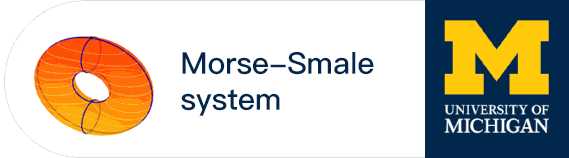#### Computation and Foundation

The theory of computation has been studied by mathematicians, logicians and computer scientists with common and different perspectives. The Godel incompleteness, Turing machine, Hilber 10th Problems are a few of remarkable theorems/models of computation/problems. At the same time, there are still many unsolved problems in this area. One of which is P =NP? in computational complexity. From 1980s, Smale and his co-workers proposed BSS computation model which can be used to study the algorithms in variety of fields, especially in numerical analysis and scientific computation. Their research results were later combined in the book “Complexity and Real Computation”. Smale Institute will continue the research in this direction with the emphasis in the algorithms in the area of data science and artificial intelligence.

Principle Investigator
Prof. Mike Shub